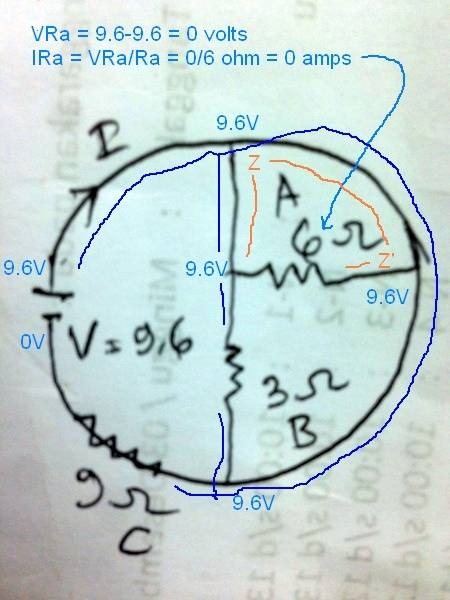# Find the current I in this electrical circuit

View attachment 216636

Is the 6o hm (A) short circuit (jumper wire)?
Let's ask this in another way. Is the voltage the same at both sides of the resistor?

Start at the positive battery terminal, and trace the circuit with your finger ...
Label the 6 ohm resistor "RA", one side of the resistor Z, and the other side Z'.
What is the voltage at Z?
9.6 volts.

And at Z'?
Also, 9.6 volts.What does Ohm's law tell us? If the voltage is the same at both sides of the resistor (that is, voltage drop across the resistor is zero) then IRA=VRA/RA = 0 volts/6 ohms = 0 amps.

In effect, 6 ohm resistor RA is shorted by the "wire" between point Z and Z'.

In this drawing it's the same for connections between RA and RB. The only component with a voltage drop across it is the 9 ohm resistor RC.

Let's re-state the problem in yet another way.
RA is a 6 ohm resistor in parallel with a 0 ohm wire between point Z and Z'.
The general rule is that total resistance for two resistors in parallel is less than the smaller resistor. In this special case the smaller resistor is 0 ohms, and limits total resistance to 0 ohms.

#### Attachments

•Helly123
Thank you for the explanation :)

In this drawing it's the same for connections between RA and RB. The only component with a voltage drop across it is the 9 ohm resistor RC.

So both A and B are short circuit, because the voltage stream in left side and right side are zero. I see.